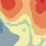# What summary statistics are available for geoanalytics.summarize_data.aggregate_points?

547
1
02-08-2017 07:52 AMNew Contributor II

Specifically, is it possible to get percentile cutoffs and CoV?  Can one define a custom statistic?

Tags (4)
1 Solution

Accepted SolutionsbyEsri Contributor

Hi Jill,

It’s not currently possible to calculate the percentile cutoffs or calculate a custom statistic during a tool run using GeoAnalytics (at 10.5).

You can calculate the CV on your GeoAnalytics results if you use two steps. First use GeoAnalytics to aggregate or summarize your data (such as running Aggregate Points). Once your data has been aggregated or summarized, you could calculate the CV using the standard analysis tools field calculator (standard deviation and mean can be calculated in the GeoAnalytics tool run).

For most GeoAnalytics tools, the statistics available are count, sum, min, max, mean, range, standard deviation and variance. You can learn more about the tools in the REST API help or Pro help.

We'll start to think about ways that we can incorporate your other suggestions into future releases of GeoAnalytics.byEsri Contributor

Hi Jill,

It’s not currently possible to calculate the percentile cutoffs or calculate a custom statistic during a tool run using GeoAnalytics (at 10.5).

You can calculate the CV on your GeoAnalytics results if you use two steps. First use GeoAnalytics to aggregate or summarize your data (such as running Aggregate Points). Once your data has been aggregated or summarized, you could calculate the CV using the standard analysis tools field calculator (standard deviation and mean can be calculated in the GeoAnalytics tool run).

For most GeoAnalytics tools, the statistics available are count, sum, min, max, mean, range, standard deviation and variance. You can learn more about the tools in the REST API help or Pro help.

We'll start to think about ways that we can incorporate your other suggestions into future releases of GeoAnalytics.